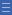## Featured resource

Home > Topdrawer > Mental computation > Activities > Splitting arrays

# Splitting arrays

Splitting arrays

In this activity, students construct a rectangular array for a given number which they then partition into areas using known facts. This assists them to derive new facts.

Partitioning or splitting arrays into known parts assists students to develop fluency and flexibility with basic number facts. It also introduces students to the distributive property.

Students work in pairs.

• One student generates two single-digit numbers by rolling 2 ten-sided dice (or two six-sided dice with faces of 4, 5, 6, 7, 8, 9 and 5, 6, 7, 8, 9, 10).
• The student records a fact (e.g. 6 $$\times$$ 7) and constructs a partial or complete array.
• The partner looks for other related facts by splitting the array (e.g. 6 $$\times$$ 5 + 6 $$\times$$ 2).

You can watch the Splitting Arrays video.

Students work together to find another way to split the array using known facts.

Opportunities arise for noticing square numbers, and doubling and halving connections. This is a useful way to develop flexibility and strategic choice. You can watch the slide show on splitting arrays into known parts.

A blank 10 by 10 grid can be downloaded and used to record the splits. Using different colours makes the connections clear.

Encourage students to generate a list of multiplication facts that they find difficult, and consider how they might partition them to make the mental calculation easier.

Make a class chart of the different strategies.

This activity can be extended so students mentally calculate the product of a two-digit and a one-digit number using partitioning strategies to make the calculation easier.

Yes

Yes

Name Class SectionYear 4: Recall multiplication facts up to 10 x 10 and related division facts Infobox 3Year 3: Recall multiplication facts of two, three, five and ten and related division facts Infobox 3Source Infobox 3